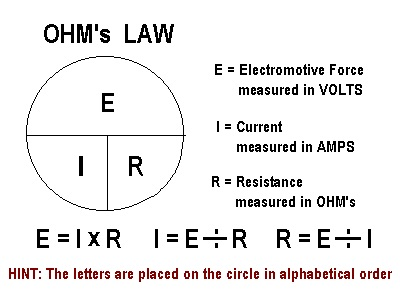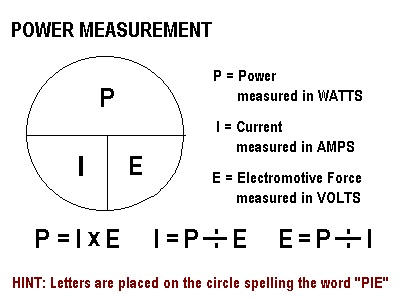# Ohms Law

The ohm, Ω, is the standard unit of resistance for direct current, and the standard unit of impedance for alternating current. According to Ohm's law, one ohm is the resistance between two points that requires one volt of potential difference to produce one ampere of current. In the process, one watt of energy is converted into heat.

Ohm's law, which states that current is inversely proportional to resistance for a given voltage. This means the current decreases as the resistance increases, or it increases as the resistance decreases.

History of the Ohm
The ohm was formally defined at an international conference in 1881, and is named after Georg Simon Ohm (1787 - 1854), a French physicist. The symbol is the Greek capital letter Omega, Ω.Ohm's LawOhm's Law